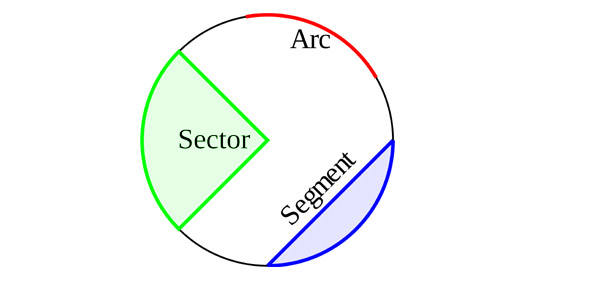# Circles Circular Logic Quiz

8 Questions | Total Attempts: 2250Settings• 1.
What do you call a line that connects 2 points on the circumference, that is not a diameter?
• A.

Diameter

• B.

Tangency line

• C.

Chord

• 2.
What do you call the point where the tangency line meets the circumference?
• A.

Point of tangency

• B.

Tangency point

• C.

None of the above

• 3.
If the radius of a circle is 10 what is the diameter?
• A.

21

• B.

20

• C.

5

• 4.
If the diameter of a circle is 80 what is the radius?
• A.

40

• B.

160

• C.

180

• 5.
There can be 2 points of tangency
• A.

True

• B.

False

• 6.
There can be more then one chord
• A.

True

• B.

False

• 7.
A diameter is a chord
• A.

True

• B.

False

• 8.
If a radius is 8 the diameter is 4
• A.

True

• B.

False

Related TopicsBack to top